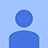# Đề thi Olympic Tiếng Anh lớp 9 vòng 2 năm 2013 - 2014

1 93

## Đề thi Olympic Tiếng Anh lớp 9 vòng 2

Đề thi Olympic Tiếng Anh (IOE) lớp 9 vòng 2 năm học 2013 - 2014 có đáp án kèm theo được VnDoc sưu tầm, chọn lọc giúp các em học sinh ôn tập và củng cố kiến thức, nhằm đạt kết quả cao trong các vòng tiếp theo của cuộc thi Olympic giải Tiếng Anh qua mạng năm học 2013 - 2014. Sau đây mời các em cùng tham khảo.

Đề thi Olympic Tiếng Anh lớp 9 vòng 1 năm 2013 - 2014

### Đề thi Olympic Tiếng Anh lớp 9 vòng 2 năm 2013 - 2014

Chọn câu trả lời tốt nhất cho những câu hỏi dưới đây.

1. A state game warden has been observing deer during a particularly severe winter. She recorded that 14 of the 96 deer she observed throughout the state appeared to be suffering from malnutrition. The state has an estimated deer population of 600,000. Based on her observations, approximately how many deer would she predict are suffering from malnutrition?

A. 14,000 B. 72,000 C. 88,000 D. 96,000 E. 15,0002. In the figure, lines A and B are straight and parallel to each other. Which angles must be congruent to ∠1?

A. ∠2 and ∠6

B. ∠4, ∠6, and ∠7

C. ∠3 and ∠5

D. ∠4, ∠5, and ∠8

E. only ∠6

3. Ms. Patrick wrote the fractions 0.75/1 , 1.5/2 , 3/4 , 9/12 on the board. Then she asked Sabrina to describe the relationship among the numbers. Which statement describes the relationship among the numbers?

A. The fractions are equivalent.
B. The fractions are in ascending order.
C. The fractions are in descending order.
D. The fractions are doubling from left to right.
E. None of them4. The table shows how many pages are left to copy at different times. How many pages are left to copy at the end of 40 seconds?

A. 11 B. 13 C. 16 D. 33 E. 55

5. An economist used the equation y = 0.05x2 + 3.63x + 37.68 to model changes in consumer prices. For a given value of y, which method should be used to solve the equation for x?

A. distance formula B. factoring

C. Pythagorean theorem D. quadratic formula

E. None of them

6. Jeremy works at a bookstore. He gets paid \$30 per day and \$2 for every book he sells. He determines his total daily pay, D, with the equation D = 30 + 2b, where b is the number of books he sells On Monday, Jeremy's total daily pay is \$50. On Tuesday, Jeremy's total daily pay will change to \$35 per day and \$2 for every book he sells. Which statement is true about Jeremy's total daily pay on Tuesday?

A. It will be \$5 more than on Monday.
B. It will be \$35 more than on Monday.
C. It might be less than on Monday.
D. It might be less than \$35.
E. None of them7. Ice cream vendors at the county fair gathered data about the various ages of people and their preferences for different ice cream flavors. The data are summarized in the table. And the circle graph represents the flavor preferences for one of the four age groups. Which age group's preferences are represented in the graph?

A. under 12 B. 12 to 20 C. 21 to 45 D. over 45 E. None of them

8. Which expression is equivalent to 812/83?

A. 19 B. 84 C. 89 D. 512 E. 152

9. Melanie paddled her kayak for 1.5 hours. She paddled 6 miles. How many miles per hour did Melanie paddle?

A. 0.25 mile per hour B. 4 mile per hour

C. 4.25 mile per hour D. 9 mile per hour

E. 14 mile per hour

10. A sphere had a 6-inch radius (r). What was the volume of the sphere?

A. 24π cubic inches B. 216π cubic inches C. 32π cubic inches

D. 288π cubic inches E. 5π cubic inches

## Đáp án đề thi Olympic Tiếng Anh lớp 9 vòng 2

Câu 1: C Câu 2: D Câu 3: A Câu 4: C Câu 5: D

Câu 6: C Câu 7: B Câu 8: C Câu 9: B Câu 10: D

Đánh giá bài viết
1 93
0 Bình luận
Sắp xếp theo